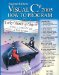# B.2. Abbreviating Binary Numbers as Octal and Hexadecimal Numbers

The main use for octal and hexadecimal numbers in computing is for abbreviating lengthy binary representations. Figure B.7 highlights the fact that lengthy binary numbers can be expressed concisely in number systems with higher bases than the binary number system.

Figure B.7. Decimal, binary, octal and hexadecimal equivalents.

Decimal number

Binary representation

Octal representation

0

0

0

0

1

1

1

1

2

10

2

2

3

11

3

3

4

100

4

4

5

101

5

5

6

110

6

6

7

111

7

7

8

1000

10

8

9

1001

11

9

10

1010

12

A

11

1011

13

B

12

1100

14

C

13

1101

15

D

14

1110

16

E

15

1111

17

F

16

10000

20

10

A particularly important relationship that both the octal number system and the hexadecimal number system have to the binary system is that the bases of octal and hexadecimal (8 and 16 respectively) are powers of the base of the binary number system (base 2). Consider the following 12-digit binary number and its octal and hexadecimal equivalents. See if you can determine how this relationship makes it convenient to abbreviate binary numbers in octal or hexadecimal. The answer follows the numbers.

 Binary number Octal equivalent Hexadecimal equivalent 100011010001 4321 8D1

To see how the binary number converts easily to octal, simply break the 12-digit binary number into groups of three consecutive bits each and write those groups over the corresponding digits of the octal number as follows:

```100 011 010 001
4 3 2 1
```

Note that the octal digit you have written under each group of three bits corresponds precisely to the octal equivalent of that 3-digit binary number, as shown in Fig. B.7.

The same kind of relationship can be observed in converting from binary to hexadecimal. Break the 12-digit binary number into groups of four consecutive bits each and write those groups over the corresponding digits of the hexadecimal number as follows:

```1000 1101 0001
8 D 1
```

Notice that the hexadecimal digit you wrote under each group of four bits corresponds precisely to the hexadecimal equivalent of that 4-digit binary number as shown in Fig. B.7.Visual C# 2005 How to Program (2nd Edition)
ISBN: 0131525239
EAN: 2147483647
Year: 2004
Pages: 600